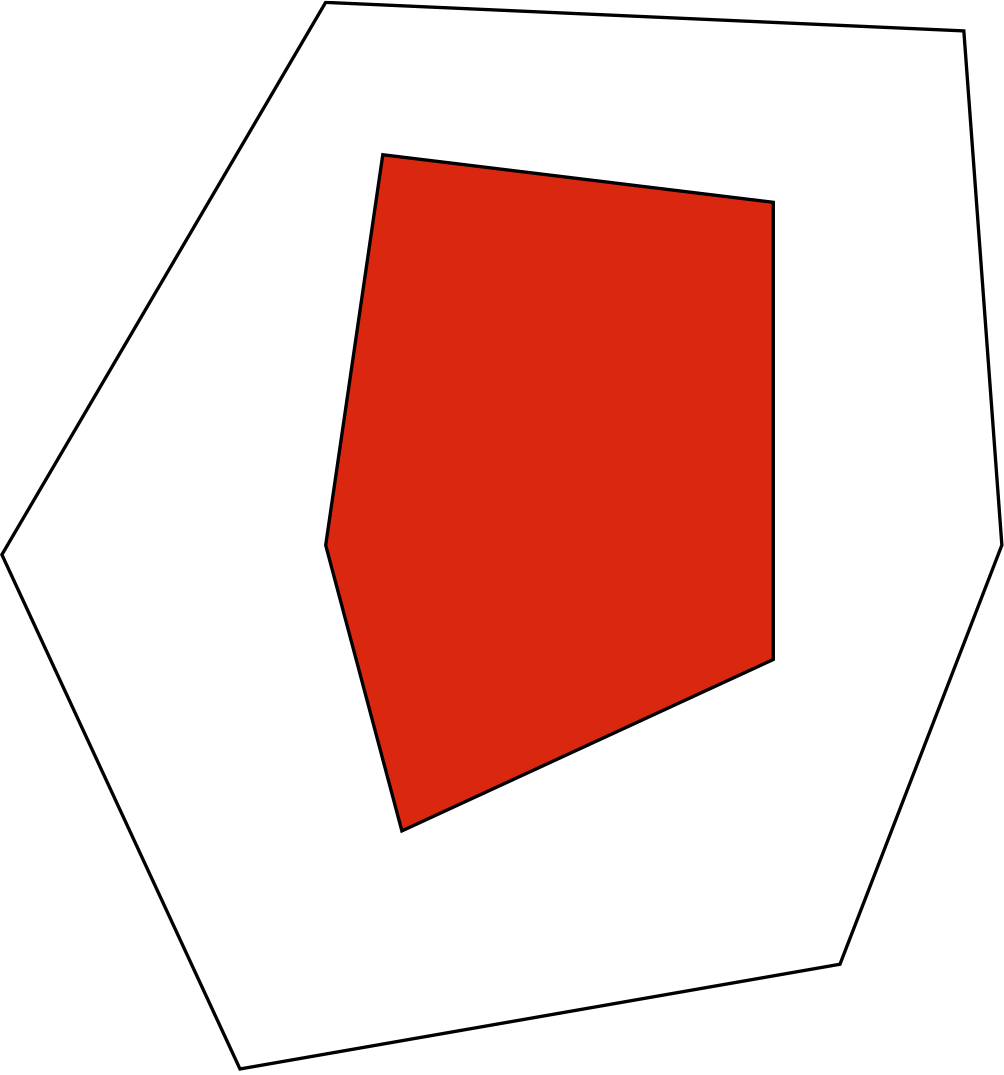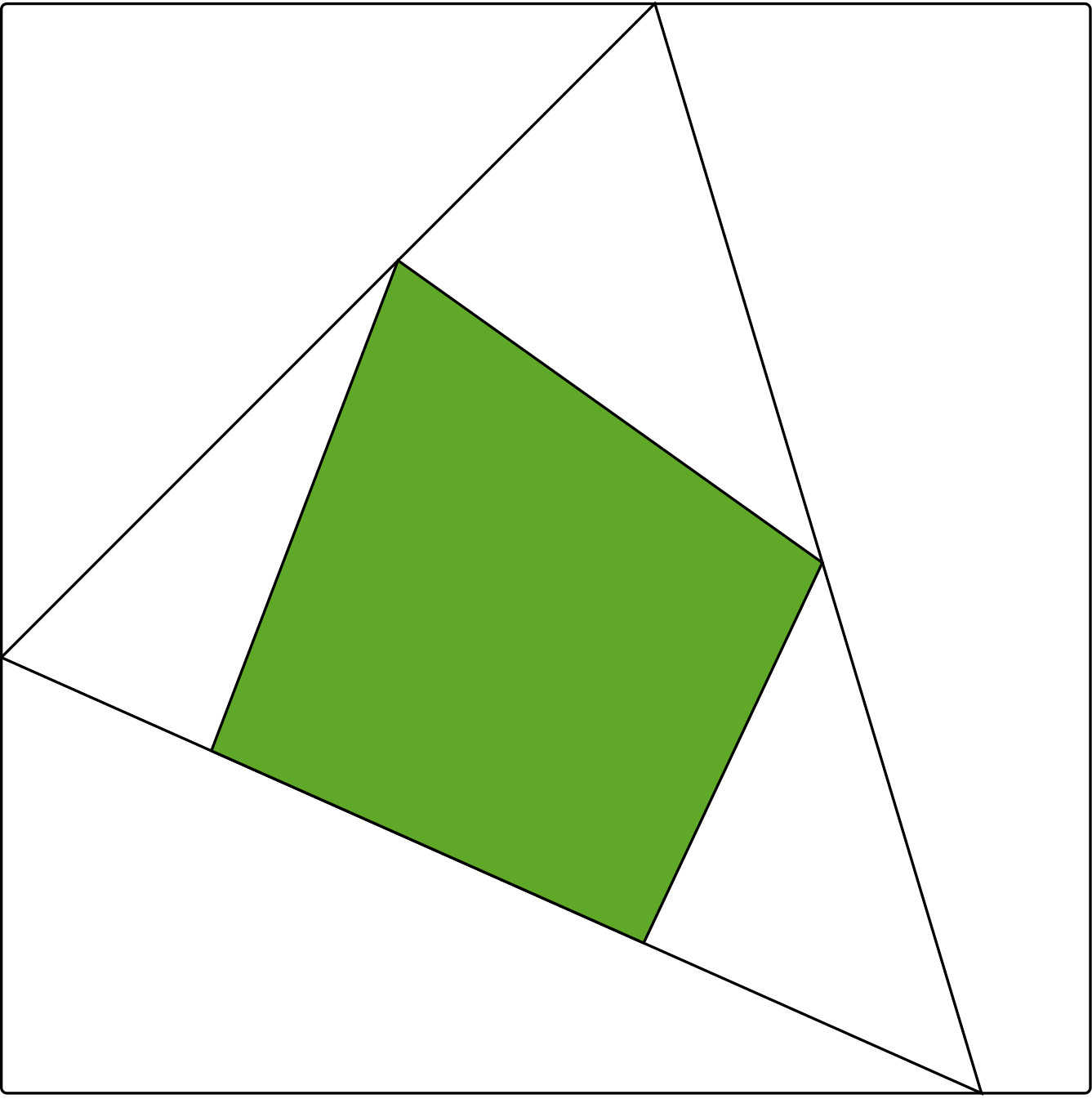# Historical talks

During the summer program on cryptography, every Monday afternoon there is a talk on the history of a classic paper or series of papers. Last week, Russell Impagliazzo spoke on his work with Steven Rudich on the impossibility of basing one-way permutations or key agreement on the existence of one-way functions. The week before, Umesh Vazirani spoke on quantum computing, and earlier Ron Rivest spoke about the origins of modern cryptography.

All talks are recorded, and the recordings are available here.

Tomorrow afternoon, Daniele Micciancio will speak at 3pm on lattice-based cryptography.

Tomorrow is also the first day of the Workshop on the mathematics of modern cryptography. The program starts at 9:30am Pacific time, and all talk are broadcast live, as usual.

# Tune in at 9:30am (12:30pm Eastern)

This Summer, most of the theory of cryptography community is in Berkeley to participate in the Simons Institute program on cryptography.

The program started with week-long series of lectures, all available here, which covered tools such as lattice problems, multilinear maps, oblivious RAMs, scrambled circuits, and differential privacy, and their applications to homomorphic encryption, obfuscation, delegated computations, and multiparty computations.

This week there is a workshop on secure computations, all whose talks are livestreamed, which starts today at 9:30 Pacific Time with a talk by Amit Sahai on obfuscation.

# Research Vignette: Semialgebraic Geometry of Ranks

By Kaie Kubjas

The rank of a matrix M is a fundamental notion in linear algebra. While usually defined as the dimension of the span of the rows or the span of the columns, it could also be defined as the minimal number r such that the matrix M admits a factorization as M=AB, where A has r columns and B has r rows. If all entries of the matrix M are nonnegative, then such a factorization can be pictured as a pair of nested polytopes: the outer one is given by the inequalities Ax≥0, and the inner one by the convex hull of the columns of B. Up to an affine transformation, this picture is independent of the specific factorization AB.Figure 1: Two nested polygons corresponding to a rank 3 matrix with 6 rows and 5 columns

In many applications, we are interested in factorizations of a certain particular form. For example, we can study factorizations M=AB where A,B have nonnegative entries. The minimal r as above for which such a factorization exists is called the nonnegative rank. Cohen and Rothblum formulated this definition geometrically: a nonnegative matrix M has nonnegative rank at most r if and only if there exists a polytope with r vertices that can be fitted between two nested polytopes associated with the matrix.Figure 2: Two quadrangles and a triangle between them

The interest in the nonnegative rank started in the combinatorial optimization community with the work of Yannakakis at the end of the 1980s. A linear program aims to minimize or maximize a linear function over a region that is given by linear constraints, i.e., a polyhedron or a polytope. Yannakakis showed that the minimal number of variables and constraints needed to express a linear program over a polytope is closely related to the nonnegative rank of a matrix associated to this polytope.

One line of research that started with the seminal paper of Yannakakis studies lower bounds on the nonnegative rank, and later also on the positive semidefinite rank, for different polytopes that appear in combinatorial optimization. For example, the Traveling Salesman Problem (TSP) asks: Given a list of cities, what is the shortest cycle that visits every city exactly once? It can be formulated as a linear program over a region which is called the TSP polytope. The TSP problem is NP-hard, and some of the attempts to prove P=NP aimed to give polynomial formulations of the TSP polytope. This motivated Yannakakis to look for superpolynomial lower bounds on the nonnegative rank of the TSP polytope, which was completed by Fiorini et al. in 2012.

The notion of nonnegative rank also appears in statistics: The set of stochastic matrices of nonnegative rank at most r is called the r-th mixture model. It represents the joint probabilities of two random variables that are independent given a third random variable with r possible values. Given a data matrix that is obtained by an opinion poll or a measurement, one would like to estimate the parameters of the true probability distribution that the data come from. Specifically, the maximum likelihood estimate of a data matrix is a matrix in the r-th mixture model that maximizes a specific function, called the likelihood function. There are several ways for numerically solving the maximum likelihood estimation in practice. These methods, however, do not provide a certificate for having found the global optimum.

In my recent research together with Eggermont, Horobeţ, Robeva, and Sturmfels (Robeva and Sturmfels attended the Algebraic Geometry program, and Horobeţ participated in one of the workshops at the Simons Insitute), we have been interested in exact descriptions of the sets of matrices of nonnegative rank at most r. They are semialgebraic sets, which means that they can be characterized by Boolean combinations of polynomial equations and inequalities. Knowing quantifier-free semialgebraic descriptions of these sets would give an exact method for checking if a matrix lies in them. It would be also an essential step towards computing maximum likelihood estimates with certificate.

# Research Vignette: Faster Algorithms for Linear Programming

By Yin Tat Lee and Aaron Sidford

## Interior Point Methods and Algorithmic Spectral Graph Theory

Linear programming has long stood as one of the key pillars of computer science. In practice, problems are often reformulated as linear programs to take advantage of fast implementations of linear programming algorithms. And in theory, the best running times for solving many problems are achieved by using the theoretical guarantees of these algorithms. Moreover, new algorithms for linear programming have tended to cause ripples across the field, yielding not just faster running times but new algorithmic frameworks and a new lens on the structure of difficult problems.

Recently, state-of-the-art linear programming techniques known as interior point methods have been making waves in the area of Algorithmic Spectral Graph Theory, which uses linear algebraic properties of graphs to improve algorithm design. Like many iterative methods for optimization, interior point methods reduce solving difficult problems, like linear programming, to solving a sequence of simpler problems — in this case, solving a system of linear equations. When solving classic network optimization problems like maximum flow and minimum cost flow using interior point algorithms, these linear systems are highly structured. To solve them, it suffices to solve linear systems in the combinatorial Laplacian of a graph, one of the central mathematical objects in spectral graph theory. Consequently, standard interior point methods provide an algorithmic framework that naturally facilitates the leveraging of spectral properties of graphs to obtain fast running times for network optimization problems.

Over the past decade, studying such problems at this boundary of continuous and discrete optimization has been an incredibly fruitful area of research. Building off work of Vaidya in 1991, in 2004 Spielman and Teng produced the first algorithm to solve these Laplacian systems in time nearly-linear in the size of the associated graph. This result influenced a decade of research in algorithmic spectral graph theory, prompted numerous breakthroughs in combinatorial optimization, and ultimately helped inspire much of the research in the Simons Institute’s Fall 2014 program on Algorithmic Spectral Graph Theory.

Moreover, Spielman and Teng’s result implied that iterations of standard interior point methods can be implemented in nearly linear time when solving common network optimization problems. In 2008, Daitch and Spielman proved that such an algorithm not only nearly matches the running time of the state-of-the-art 1998 algorithm of Goldberg and Rao for solving the maximum flow problem, but actually improves upon the previous best known algorithms for solving the minimum cost flow problem. Even more excitingly, over the past two years, modified interior point schemes and fast Laplacian system solvers have been used to improve the running time for exactly solving the maximum flow problem. In 2013, Aleksander Madry provided a faster algorithm for solving sparse unit capacity instances of maximum flow, and in 2014 we provided a new interior point scheme with a faster convergence rate that yielded faster algorithms for solving dense instances of the maximum flow and the minimum cost flow problems.

This interplay between algorithms for continuous optimization and the discrete structure of graphs was one of the central themes of the Simons Institute’s Fall 2014 Program on Algorithmic Spectral Graph Theory. Interior point methods and fast Laplacian system solvers are simply one illustration. Both in recent years and during the program there has been extensive work on using new iterative machinery and new structural properties of graphs to design faster and better algorithms for solving various graph problems. During the semester we enjoyed exploring multiple research questions in this direction and discussing the exciting work of visitors and long term participants. In the remainder of this vignette we will discuss one recent success story in this line of work.